Libri di Angiola Pollastri - libri Statistica & Applicazioni

# Angiola Pollastri

### Author's titles

Distribution of the ratio of female income over male income digital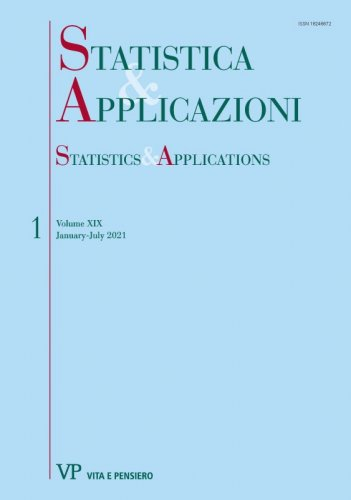Year: 2021
There is a great discussion concerning the differences between the income of females and males. The purpose of the present study is to estimate the distribution of the ratio of female income over male income. The methodology to study the ratio in exam is based on the distribution of the ratio of two Dagum random variables with three parameters proposed in 2010 by Pollastri and Zambruno...
Asymptotic Confidence Intervals for Parameters Estimated through the Ratio of Asymptotically Normal Statistics digital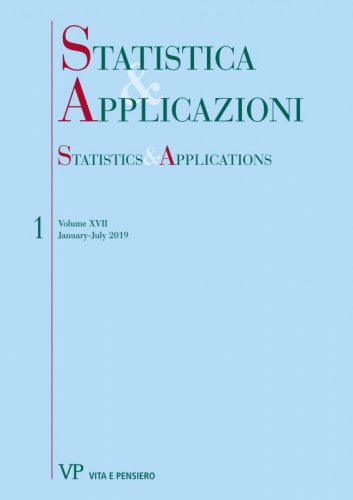Year: 2019
In this paper, four different approaches for the definition of asymptotic confidence intervals for the ratio of two unknown parameters are reviewed and compared via a simulation study. The considered approaches are based on the well known Delta Method and on the distribution of the ratio of correlated normal random variables...
The distribution of the absolute value of the ratio of two correlated normal random variables digital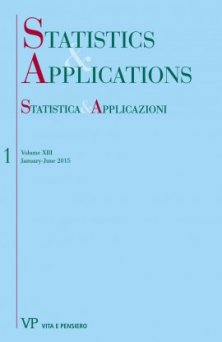Year: 2015
The aim of this paper is to study the distribution of the absolute quotient of two Correlated Normal random variables (r.v.s). This study may have many applications, as often the researcher expects a ratio to be positive or considers the sign of the ratio unimportant...
Testing for preference change in marketing or opinion research
Free
digital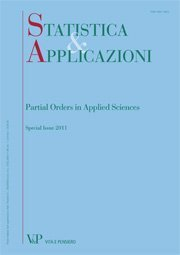Year: 2012
SUMMARY Let us suppose we have two important products or parties or opinions indicated respectively by A and B and a pool of small other choices indicated by C and we are interested in understanding if the percentage of preferences for A and the percentage of preferences for B are unchanged, increased or decreased after some event (f.i. advertising). If the sample size is big, we propose to use a two stage hypotheses test proposed by Duncan (Miller, 1981) and improved by Pollastri (2008). The test considered is based on the exact distribution of the absolute maximum (Zenga, 1979) and on the exact distribution of the absolute minimum (Pollastri and Tornaghi, 2004) of the components of a Bivariate Correlated Normal. Tables of the critical values are reported. The test proposed allows to accept one of the nine hypotheses about the invariance or increasing or decreasing of the percentage of A combined with the three movements of the percentage of B. Keywords: Trinomial Distribution, Bivariate Correlated Normal, Two Stage Hypotheses Test, Absolute Maximum and Minimum.
Estimation of equivalence scales in Italy based on income distribution
Free
digital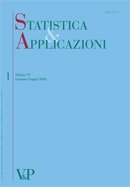Year: 2007
This paper illustrates a method for estimating equivalence scales, that is, the income required by one household to reach the same standard of living as another one. The original method considered in the present study was introduced by Kot in 2002, whereas here we formulate a different procedure based on the quantiles of the Dagum distribution. We estimate the scale parameter through the maximum likelihood method based on the results of the survey carried out by the Banca d’Italia on the household income and wealth in 2002. The reason for the chosen method is based on the fact that the Dagum distribution fits the Italian income distribution well. Finally, two ways for finding the confidence interval for the elasticity of the equivalence scale are considered.